# McDougal Littell Algebra 1 Chapter 1: Expressions, Equations & Functions Chapter Exam

Exam Instructions:

Choose your answers to the questions and click 'Next' to see the next set of questions. You can skip questions if you would like and come back to them later with the yellow "Go To First Skipped Question" button. When you have completed the practice exam, a green submit button will appear. Click it to see your results. Good luck!

### Page 1

#### Question 2 2. For these questions, I strongly recommend using either the CLEP calculator (found online) or one you use in class. Find x.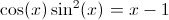#### Question 5 5. For these questions, I strongly recommend using either the CLEP calculator (found online) or one you use in class. Where is the maximum value of f(x) between 0 and pi?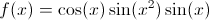### Page 3

#### Question 12 12. For these questions, I strongly recommend using either the CLEP calculator (found online) or one you use in class. What is the root of f(x)? (where f(x) is equal to zero)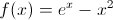### Page 4

#### Question 17 17. For these questions, I strongly recommend using either the CLEP calculator (found online) or one you use in class. Find x.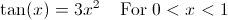### Page 5

#### Question 21 21. Which of the following is the math sentence of the following inequality?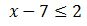### Page 6

#### Question 26 26. The following graph is which of the functions below?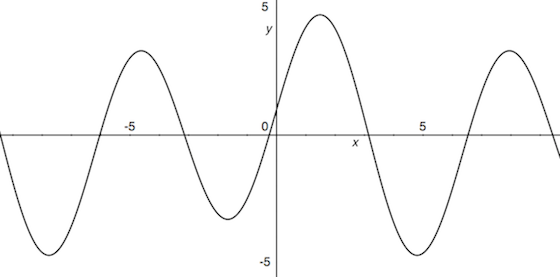#### Question 28 28. Evaluate the expression when x = -1 and y = 4.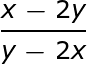#### McDougal Littell Algebra 1 Chapter 1: Expressions, Equations & Functions Chapter Exam Instructions

Choose your answers to the questions and click 'Next' to see the next set of questions. You can skip questions if you would like and come back to them later with the yellow "Go To First Skipped Question" button. When you have completed the practice exam, a green submit button will appear. Click it to see your results. Good luck!

Support